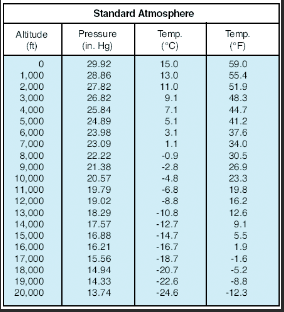Search
•CHI Aerospace

# Five Types of Altitude

Altitude is the vertical distance above a point used as a reference, but did you know there are five types of altitudes? There are many factors that play a role in determining altitude, including the vertical distance above mean sea level and above the ground surface, as well as pressure and density. Read more below to get a better understanding of the five different types of altitude, and try to get familiar with these terms to help you become a safer pilot.Indicated Altitude - This is the altitude read directly from altimeter (uncorrected) when set to the current altimeter setting.

True Altitude - The vertical distance of the aircraft above sea level. It’s often expressed in ‘MSL’ or mean sea level. Aeronautical charts use true altitude to show airports, terrain, and obstacle elevations.

Absolute Altitude - The vertical distance of an aircraft above the terrain, or above ground level (AGL).

Pressure Altitude - The altitude indicated when the altimeter setting window is adjusted to 29.92” Hg. This is the altitude above the standard datum plane, which is a theoretical plane where air pressure equals 29.92’’ Hg. ATIS tells you the value to set your altimeter and this is *very* important!

Note about the Standard Datum Plane (SDP)

The Standard Datum Plane is a theoretical level where the pressure of the atmosphere is 29.92” Hg and the weight of the air is 14.7 PSI. As the atmospheric pressure changes, the SDP also changes to be either below, at, or above sea level.Under standard conditions at sea level, the average pressure exerted by the weight of the atmosphere is about 14.70 pounds per square inch (PSI) of surface, which is 29.92” Hg. Pressure decreases with altitude; as altitude increases, the air becomes less dense. As a result, the weight of the atmosphere at 18,000 feet is half of that at sea level.

As you can see from the standard atmosphere table, atmospheric pressure changes with time and location and therefore a standard reference was developed. Standard pressure at sea level has a surface temperature of 15 degrees Celsius and a pressure of 29.92” Hg. There is a lapse rate, meaning a 2 degree Celsius drop in temperature per every 1,000 feet increase in altitude. Any temperature or pressure that differs from the standard lapse rate is considered nonstandard temperature and pressure.

And finally, there's Density Altitude - the altitude in the standard atmosphere corresponding to a particular air density value. Density altitude determines aircraft performance in the nonstandard atmosphere. It is determined by first finding pressure altitude, then correcting this altitude for nonstandard temperature variations. Density altitude is affected by altitude, temperature and humidity, all of which play a role in aircraft performance.

We hope this helps clarify some of the confusion about the five different types of altitude. If you need more practice or clarification, feel free to reach out to your CHI Aerospace flight instructor.

Images:

Sources:

https://www.faa.gov/regulations_policies/handbooks_manuals/aviation/phak/media/pilot_handbook.pdf